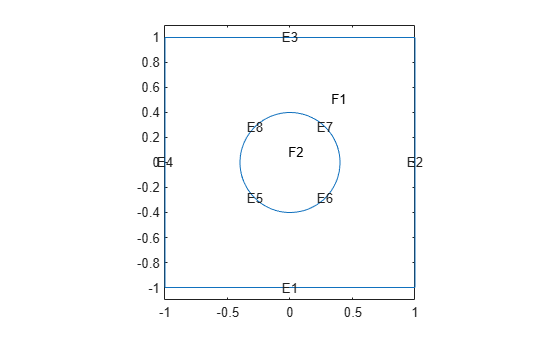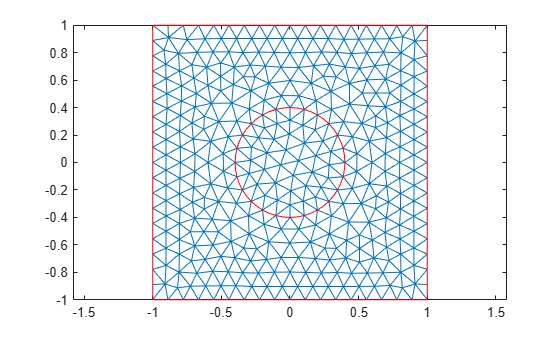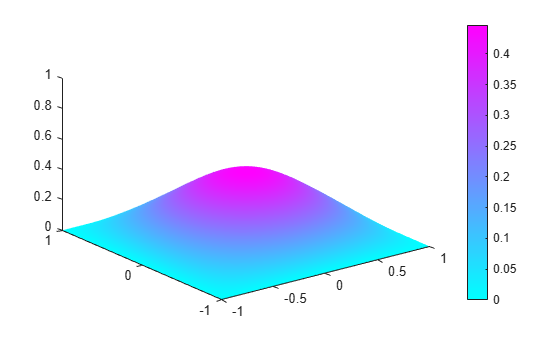Documentation

### This is machine translation

Mouseover text to see original. Click the button below to return to the English version of the page.

To view all translated materials including this page, select Country from the country navigator on the bottom of this page.

## Inhomogeneous Heat Equation on Square Domain

This example shows how to solve the heat equation with a source term.

The basic heat equation with a unit source term is

``

This is solved on a square domain with a discontinuous initial condition and zero temperatures on the boundaries.

### Create Thermal Model and Geometry

Create a transient thermal model.

```thermalmodel = createpde('thermal','transient'); ```

Create a square geometry centered at `x = 0` and `y = 0` with sides of length 2. Include a circle of radius 0.4 concentric with the square.

```R1 = [3;4;-1;1;1;-1;-1;-1;1;1]; C1 = [1;0;0;0.4]; C1 = [C1;zeros(length(R1) - length(C1),1)]; gd = [R1,C1]; sf = 'R1+C1'; ns = char('R1','C1')'; g = decsg(gd,sf,ns); ```

Append the geometry to the model.

```geometryFromEdges(thermalmodel,g); ```

### Specify Thermal Properties and Internal Heat Source

Specify thermal properties of the material.

```thermalProperties(thermalmodel,'ThermalConductivity',1,... 'MassDensity',1,... 'SpecificHeat',1); ```

Specify internal heat source.

```internalHeatSource(thermalmodel,1); ```

### Set Zero Temperatures on Boundaries

Plot the geometry and display the edge labels for use in the boundary condition definition.

```figure pdegplot(thermalmodel,'EdgeLabels','on','FaceLabels','on') axis([-1.1 1.1 -1.1 1.1]); axis equal title 'Geometry With Edge and Subdomain Labels' ```Temperature is zero at all four outer edges of the square.

```thermalBC(thermalmodel,'Edge',1:4,'Temperature',0); ```

### Specify Initial Temperature

The discontinuous initial value is 1 inside the circle and zero outside. Specify zero initial temperature everywhere.

```thermalIC(thermalmodel,0); % Specify non-zero initial temperature inside the circle (Face 2). thermalIC(thermalmodel,1,'Face',2); ```

### Generate and Plot Mesh

```msh = generateMesh(thermalmodel); figure; pdemesh(thermalmodel); axis equal ```### Find and Plot Solution

Find the solution at 20 points in time between 0 and 0.1.

```nframes = 20; tlist = linspace(0,0.1,nframes); thermalmodel.SolverOptions.ReportStatistics ='on'; result = solve(thermalmodel,tlist); T = result.Temperature; ```
```99 successful steps 0 failed attempts 200 function evaluations 1 partial derivatives 20 LU decompositions 199 solutions of linear systems ```

Plot the solution.

```figure Tmax = max(max(T)); Tmin = min(min(T)); for j = 1:nframes, pdeplot(thermalmodel,'XYData',T(:,j),'ZData',T(:,j)); caxis([Tmin Tmax]); axis([-1 1 -1 1 0 1]); Mv(j) = getframe; end ```To play the animation, use the `movie(Mv,1)` command.

##### SupportGet trial now